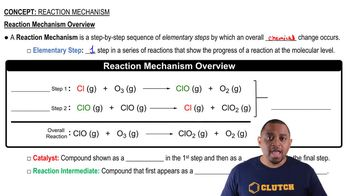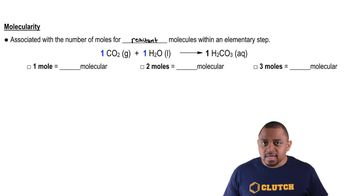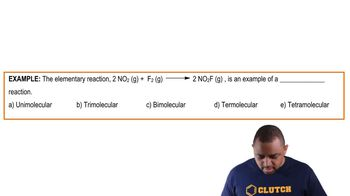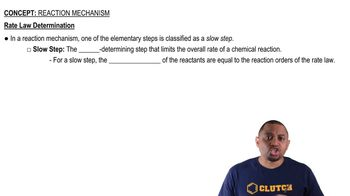Start typing, then use the up and down arrows to select an option from the list.# Reaction Mechanism Concept 2

Jules Bruno
284views
Now, Molecular charity is associated with the number of moles for reactant molecules within an elementary step. Here we take a look, we have one mole of carbon dioxide gas reacting with one mole of water liquid to produce one mole of carbonic acid. If we were to take a look here, we'd say that we have a total of two moles of reactivates. Now the number of moles of reactant gives us different names for the molecular charity. If we had only one mole of a reactant, then the molecular charity would be called uni. Molecular. If there are two moles of reactant like we have in this example, then that would be called by molecular. Now, I know when we're naming Covid and compounds in the past, when we say to we'd say die like carbon dioxide, but for Molecular charity we don't use the prefix di we use the prefix by so two moles of reactant Sequels by molecular. If we had three moles of reactant total, then that would be ter molecular. When we talked about numerical prefixes in the past three would be try but there's no such thing as try. Molecular. So again, when it's three moles of reactant, its term molecular Okay, so when we talk about molecular charity, just count the total number of reactive molecules and you'll be able to determine the molecular charity of any elementary step03:0602:1401:3101:0200:5804:16